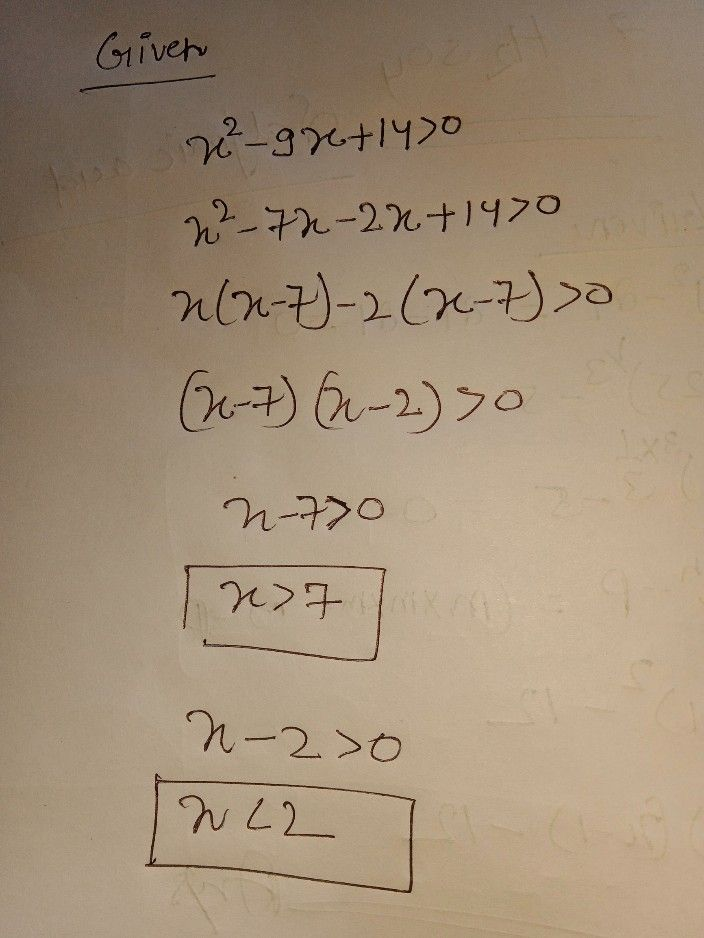Symbol
ProblemActivity $3$ $Dlroction$ $Fi11-ln$ the table below to find the solution set of each of the following quadratic inequalities. $1\right)$ $x^{2}-9x+14>0$ 0 (Use three test point $metn0d$ Write the quadratic inequality in standard form. roots of $1t9$ Find the $ra$ corresponding equality Find the $3t6$ test points Test $a$ value from each interval $1n$ the inequality. Test the roots Solution set